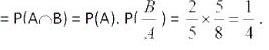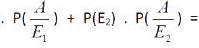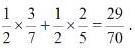UPSC  >  Probability: Solved Examples- 2

# Probability: Solved Examples- 2 - CSAT Preparation - UPSC

Example 1: An urn contains 10 white and 15 black balls. Two balls are drawn in succession without replacement. What is the probability that first is white and second is black?

Solution: Let A = Getting a white ball in the first draw B = Getting a Black Ball in the second draw. Required Probability = Probability of getting a white ball in the first draw and a black ball in second draw = P(A and B) = P (A ∩B) = P (A) .P(B/A)

P(A) = (10C1/25C1) = (10/25) = (2/5) and P(B/A) = Probability of getting a black ball in the second draw
when a white ball has already been in first draw  = (15C1/24C1) = (15/24) = (5/8)
⇒ Required probabilityExample 2: A standard die is rolled. Let C be the event that the outcome is an odd number and D be the event that the outcome is not more than 3. Find the probability C knowing that D has happened?
Solution: Let's find the conditional probability of C given that D has occurred.
If we know D has occurred, the possible outcomes are {1,2,3}.
For C to happen the outcome must be in C∩D={1,3}.
P(C|D) = P(C∩D)/P(D) = 2/3

Example3: Hunar wrote two sections of CAT paper ; Verbal and QA in the same order. The probability of her passing both sections is 0.6. The probability of her passing the verbal section is 0.8. What is the probability of her passing the QA section given that she has passed the Verbal section?

Solution: Let P(QA) = passing QA’s section. And P(V) = passing Verbal section. So, P(QA/V) = P(QA∩V)/P(V) = 0.6/0.8⇒0.75
Example 4: Amika has 2 pouches. One pouch contains 3 golden and 4 silver crystals. The second pouch contains 2 golden and 3 silver crystals. One pouch is selected at random and from the selected pouch, one crystal is drawn. Find the probability that the crystal drawn is golden.
Solution: Since one of the two pouches is selected randomly, So,P(E1) =1/2 and P(E2) = 1/2 .
Now P(A/E1) = Probability of drawing a golden crystal when the first pouch has been chosen =3/7 and P(A/E2)=Probability of drawing an golden crystal when the second pouch has been selected = 2/5
[∴ The second pouch contains 2 golden and 4 silver crystals]

Using the law of total probability P(golden crystal) = P(A) = P(E1) .Example 5: A year is selected at random. What is the probability that it contains 53 Mondays if every fourth year is a leap year?( It is a very famous question and appears frequently in many competitive exams.)
Solution: Let P(L) = probability of selecting a leap year. P(NL) = probability of selecting a non - leap year. P(M) = probability of getting 53 Mondays in a year.

Then by Law of total probability: P(M) = P (M| L) . P(L) + P (M | NL) . P(NL)

= (2/7).(1/4) + (1/7).(3/4) = 5/28

Example 6: There are three cartons, each containing a different number of soda bottles. The first carton has 10 bottles, of which four are flat, the second has six bottles, of which one is flat, and the third carton has eight bottles of which three are flat. What is the probability of a flat bottle being selected when a bottle is chosen at random from one of the three cartons?
Solution: Let A be the event that the selected bottle is flat, X be the event that it is chosen from carton 1, Y be the event that it is chosen from carton 2, Z be the event that it is chosen from carton 3.

Then by the Law of total probability:

P(A) = P(A | X)-P(X) + P(A | Y) P(Y ) + P(A/Z).P(Z).

= (4/ 10).(1/ 3) + (1/ 6) . (1/ 3) + (3/8) .(1/3) = 113/360 .

The document Probability: Solved Examples- 2 | CSAT Preparation - UPSC is a part of the UPSC Course CSAT Preparation.
All you need of UPSC at this link: UPSC

## CSAT Preparation

197 videos|151 docs|200 tests

## FAQs on Probability: Solved Examples- 2 - CSAT Preparation - UPSC

 1. What is probability and how is it calculated?Ans. Probability is a measure of the likelihood of an event occurring. It is calculated by dividing the number of favorable outcomes by the total number of possible outcomes.
 2. How can probability be used in real-life situations?Ans. Probability can be used in various real-life situations, such as predicting weather conditions, analyzing stock market trends, assessing risks in insurance, and determining the chances of winning in games of chance.
 3. What are the different types of probability?Ans. There are three main types of probability: theoretical probability, experimental probability, and subjective probability. Theoretical probability is based on mathematical calculations, experimental probability is determined through conducting experiments, and subjective probability is based on personal judgments or opinions.
 4. What is the difference between independent and dependent events in probability?Ans. In probability, independent events are those where the occurrence of one event does not affect the probability of the other event. On the other hand, dependent events are those where the occurrence of one event affects the probability of the other event.
 5. How can probability be used to make informed decisions?Ans. Probability can be used to make informed decisions by providing a quantifiable measure of uncertainty. By considering the probability of different outcomes, individuals or organizations can assess risks, evaluate potential outcomes, and make decisions based on the likelihood of success or failure.

## CSAT Preparation

197 videos|151 docs|200 testsExplore Courses for UPSC exam### How to Prepare for UPSC

Read our guide to prepare for UPSC which is created by Toppers & the best Teachers
Signup to see your scores go up within 7 days! Learn & Practice with 1000+ FREE Notes, Videos & Tests.
10M+ students study on EduRev
Track your progress, build streaks, highlight & save important lessons and more!
Related Searches

,

,

,

,

,

,

,

,

,

,

,

,

,

,

,

,

,

,

,

,

,

;